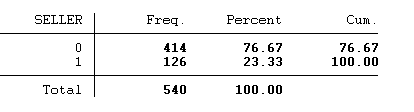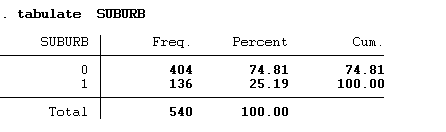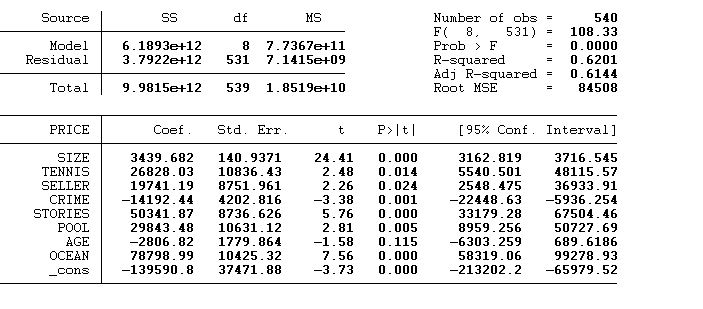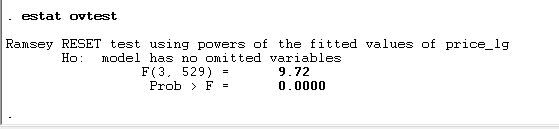## 回归模型代写 定量方法 (M), (UAC) & (H) 2021 年第 1 学期 重大项目 介绍。

Selling of houses is one of the things that has become a real challenge in todays time. In this project my main aim is to make sure that I use the available to make predictions of the price of a house based on the features related to the house. The method I am going to employ here is to use the multiple linear regression to model the price of the houses as a function of the explanatory variables. The data in use will be from a small town called Junction which has 2 suburbs.

### Data summary

The following table presents the summary statistics of the various variables;

. summarize  AGE  CRIME TOWN PRICE SIZE STORIES

### 数据汇总 回归模型代写

.总结年龄犯罪城镇价格大小故事

Variable |       Obs        Mean    Std. Dev.       Min        Max

————-+——————————————————–

AGE |       540    14.97778    2.065591          8         21

CRIME |       540    2.496296    .8689582          1          3

TOWN |       540    52.44444    13.03437         30         60

PRICE |       540    417094.2    136082.8      55000    3230000

SIZE |       540      160.45     26.6797         89        450

————-+——————————————————–

STORIES |       540    1.159259     .422695          1          4

The summary statistics above are related to the numeric variables where I will use the mean of the variables to describe them. The houses have an average age of 14.9 which is 15 years. The average crime rate of 2.5 is really high in this area. Then  our predictor variable which is the price has an average price of 417094.2 with the maximum price being 3230000 and the minimum price being 55000.

The following graph will help us understand the distribution of the dependent variable in this case I will use the histogram;

The distribution of the dependent variable is not normal in this case as we can see from the summary statistics and the graph that it rightly skewed.The table shows the frequencies of the seller that is W&M represents 0 which are the majority in this case 414 and A&B represented by 1 which shows that most houses have been sold by W&M company.Mayfair (0) seems to have many of the houses that were sold as compared to Claygate (1) this can be seen from the frequency distribution above.

### Regression model

I had to run a regression model by adding and removing variables so that I may get the variables that explain the dependent variable very well.

Starting off with regressing the price to all the variables shows that some few variables are omitted due to collinearity. These variables contain different information. And as seen they are coded as numeric variables instead of being categorical in nature. The variables also did not have a higher R squared as is required so some changes had to be made.

### 回归模型

This is the model that comprises of all the variables. And as seen it has an R-squared of 0.0261 which is way too far from the required 88%.

So, the next step is to omit some of the variables that had the issues of collinearity. And then fit the model again. After trying out different variables. And fitting the regression model again I was able to get the following model fit which comprised. Of different explanatory variables explaining a variation 62.1% of the dependent variable (price).The model still did not prove to be more predictive because the R-squared was still low. So I had to transform the dependent variable by log transforming it. Because from the distribution we saw that it was skewed so I had to make it normal. With that the model produced an R-Squared of 70.67. which represents the proportion of variance in price explained by the explanatory variables.

Therefore, my final regression model equation will a multiple one which will be;

Log_Price = 12.08674 + 0.005081*(SIZE) + 0.0454932*(TENNIS) + 0.0337884*(SELLER) – 0.0388305*(CRIME) + 0.019507*(STORIES) + 0.0278956*(POOL) + 0.0033949*(AGE) + 0.1600511*(OCEAN).

Using the regression equation above to make prediction for Kelly’s house.

Log_Price = 12.08674 + 0.005081*(SIZE) + 0.0454932*(TENNIS) + 0.0337884*(卖方) – 0.0388305*(犯罪) + 0.019507*(STORIES)*06(STORIES)*016(05)*306(09)*05.06(0.0454932*(网球)+0.0337884*(卖方)) ）。

Price = exp(12.09) + exp(0.005081)*189 + exp(0.0455)*1 + exp(0.0338)*0 + exp(-0.0388)*1 + exp(0.0195)*2 +  exp(0.0279)*1  + exp(0.00339)*9 + exp(0.16) * 1 = 178280.3575.

The 90% predictive intervals for the sale price is [408,336.3634, 411366.5726]

I will advise Kelly to use W&M because the commission is too low and also they have a reputation of selling many houses as compared to the A&B.

### Regression model – evaluation

Running a Ramsey 1969 RESET test ( Regression Specification-Error Test) which checks for misspecification in the linear regression model.

### 回归模型——评估 回归模型代写After running the test and with 0.05 significance level I will reject the null hypothesis. And conclude that we have some omitted variables. The next will be to test for non-linearity in the model.

Again, using a significance level of alpha = 0.05 I get a significant result which means we have non-linearity in the model. What I did to deal with the mis specified part is I log transformed the outcome variable. And dropped some variables which were correlated. By log transforming the outcome variable it improved the proportion of variance that was explained. By the independent variables on the price, dropping the corelated variables also helped to increase the R-Squared. The data set had some issues that is it did not indicate if the variables were categorical. Or not and this really posed a problem for the model to identify the relationships very well and for making the predictions.

The data set available could not be used to make a better model that can be used to predict the sale price. This is because it had several issues that can not be modeled by the model well. So what I can mention is to say that the Kelly can prefer using different. And better models that can learn well from the data. With a better model we can have better predictions.QQ在线咨询

QQ:3554475127

QQ:3042439236